# NR Cell Performance Evaluation with MIMO

This example models a 5G New Radio (NR) cell with multiple-input multiple-output (MIMO) antenna configuration and evaluates the network performance. You can customize the scheduling strategy to leverage the downlink MIMO capabilities and analyze the performance. The example performs measurement on multi-port channel state information reference signals (CSI-RS) which gNB uses to make MIMO scheduling decisions.

### Introduction

MIMO improves network performance by improving the cell throughput and reliability. The example performs layer mapping and precoding to utilize MIMO in the downlink (DL) direction. The CSI report sent by UEs serves as an input to take MIMO scheduling decisions.

The example considers these operations within gNB and UEs that facilitate DL transmission and reception.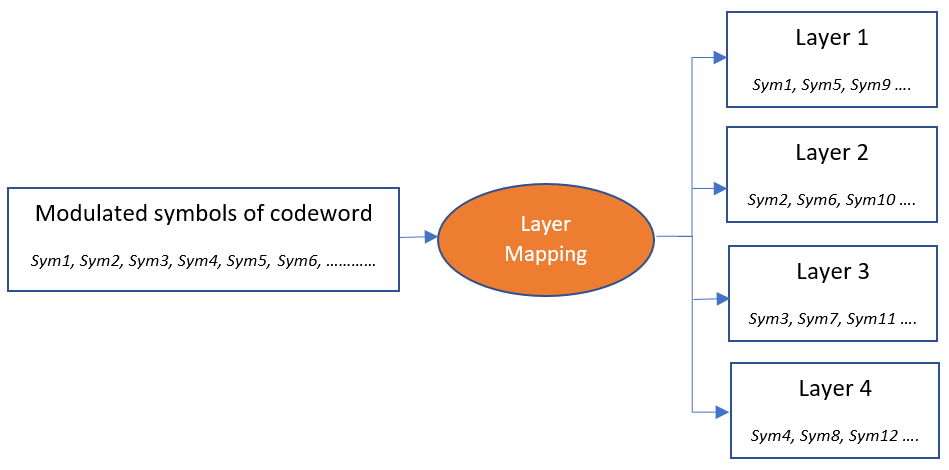This example models:

• Single-codeword DL spatial multiplexing to perform multi-layer transmission. Single-codeword limits the number of transmission layers to 4.

• DL precoding to map the transmission layers to antenna ports. The example assumes one-to-one mapping from antenna ports to physical antennas.

• DL channel quality measurement by UEs based on the multi-port CSI-RS received from the gNB. The same CSI-RS configuration applies to all the UEs.

• Precoding matrix indicator (PMI) and channel quality indicator (CQI) reporting by UEs. The example does not support rank estimation and provides the rank to be reported by a UE as a configuration parameter. A UE reports the PMI and CQI with respect to the configured rank. The example supports Type-1 single-panel codebook for PMI.

• PDSCH demodulation reference signal (DM-RS).

• Free space path loss (FSPL), additive white Gaussian noise (AWGN), and clustered delay line (CDL) propagation channel model.

Nodes send the control packets (DL assignment, PDSCH feedback, and CSI report) out of band, without the need of resources for transmission and assured error-free reception.

### DL MIMO

These are the key aspects of DL-MIMO.

#### Spatial multiplexing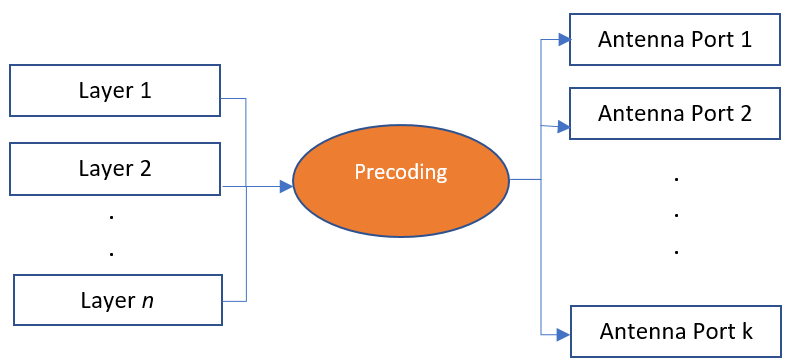Spatial multiplexing utilizes MIMO to perform multi-layer transmission. The minimum of number of transmit and receive antennas limits the number of layers (or maximum rank). The layer mapping process maps the modulated symbols of the codeword onto different layers. It maps every ${\mathit{n}}_{}^{\mathrm{th}}$ symbol of the codeword to ${\mathit{n}}_{}^{\mathrm{th}}$ layer. For instance, this figure shows the mapping of a codeword onto four layers.

#### PrecodingPrecoding, which follows the layer mapping, maps the transmission layers to antenna ports. Precoding applies a precoding matrix to the transmission layers and outputs data streams to the CSI-RS antenna ports.

#### CSI reporting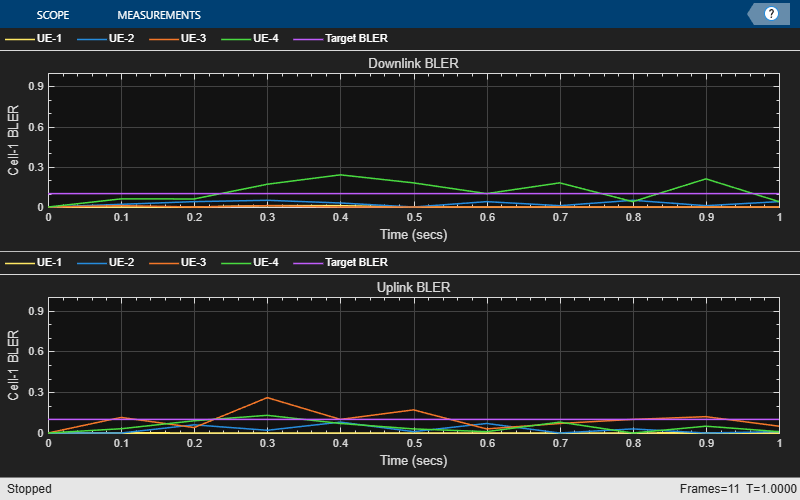CSI reporting is the process by which a UE, for DL transmissions, advises a suitable number of transmission layers (rank), PMI, and CQI values to the gNB. The UE estimates these values by performing channel measurements on its configured CSI-RS resources. For more details, see the 5G NR Downlink CSI Reporting example. The gNB scheduler uses this advice to decide the number of DL transmission layers, precoding matrix, modulation and coding scheme (MCS).

### NR Protocol Stack

A node (gNB or UE) is a composition of NR stack layers. The helper classes hNRGNB.m and hNRUE.m create gNB and UE nodes, respectively, containing the radio link control (RLC), medium access protocol (MAC), and physical layer (PHY). For more details, see the NR Cell Performance Evaluation with Physical Layer Integration example.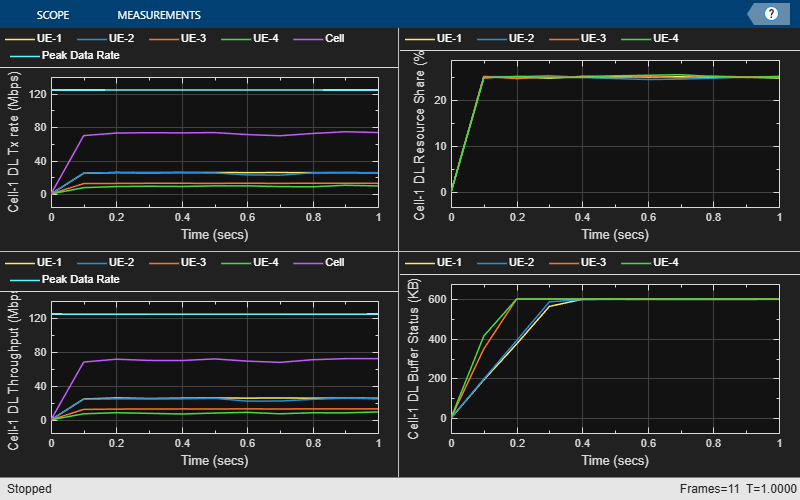### Scenario Configuration

Configure simulation parameters in the `simParameters` structure.

```rng('default'); % Reset the random number generator simParameters = []; % Clear the simParameters variable simParameters.NumFramesSim = 10; % Simulation time in terms of number of 10 ms frames simParameters.SchedulingType = 0; % Set the value to 0 (slot based scheduling) or 1 (symbol based scheduling)```

Specify the number of UEs in each cell, assuming that UEs have sequential radio network temporary identifiers (RNTIs) from `1` to `simParameters.NumUEs`. If you change the number of UEs, ensure that these simulation parameters are arrays of length equal to the value of `simParameters.NumUEs`: `simParameters.UEDistance`, `simParameters.DLPacketPeriodicityUEs`, `simParameters.DLPacketSizesUEs`, `simParameters.UERxAnts`.

```simParameters.NumUEs = 4; simParameters.UEDistance = [450; 600; 1000; 1500]; % Validate the distance of UEs from the gNB validateattributes(simParameters.UEDistance, {'numeric'}, {'nonempty', 'vector', 'finite', 'numel', simParameters.NumUEs, '>', 0}, 'simParameters.UEDistance', 'UEDistance');```

Specify the antenna counts at the gNB and UEs.

```simParameters.GNBTxAnts = 16; simParameters.UERxAnts = [2; 2; 2; 2];```

Set the channel bandwidth to 10 MHz and the subcarrier spacing (SCS) to 15 kHz as defined in 3GPP TS 38.104 Section 5.3.2.

```simParameters.NumRBs = 52; simParameters.SCS = 15; % kHz simParameters.DLBandwidth = 10e6; % Hz simParameters.DLCarrierFreq = 2.635e9; % Hz```

Specify the CSI-RS configuration.

```simParameters.CSIRSRowNumber = 11; % CSI-RS row number as per 3GPP TS 38.211 Table 7.4.1.5.3-1 simParameters.CSIRSSubcarrierLocation = [1 3 5 7]; simParameters.CSIRSSymbolLocation = 0; simParameters.CSIRSPeriod = [10 2]; % Slot periodicity and offset```

Specify the CSI report configuration.

```simParameters.PMIMode = 'Subband'; % 'Wideband' or 'Subband' simParameters.CQIMode = 'Subband'; % 'Wideband' or 'Subband' simParameters.PanelDimensions = [8 1]; % [N1 N2] as per 3GPP TS 38.214 Table 5.2.2.2.12 simParameters.SubbandSize = 4; % Refer TS 38.214 Table 5.2.1.4-2 for valid subband sizes simParameters.CodebookMode = 1; % 1 or 2```

Set the rank to be advised by UEs in CSI report. The example does not support rank estimation so the UEs use this rank to advice suitable PMI and CQI. For each UE, set a number less than or equal to the minimum of gNB's transmit antennas and UE's receive antennas. This is just the advised rank and you can customize the gNB scheduler to use a different rank for PDSCH transmission.

`simParameters.RankIndicator = [2 2 2 2]; `

Specify the signal-to-interference-plus-noise ratio (SINR) to a CQI index mapping table for a block error rate (BLER) of 0.1.

```simParameters.SINR90pc = [-7.46 -2.46 2.54 7.05 9.54 12.04 13.54 16.04 ... 18.04 20.43 22.93 23.43 25.43 28.43 31.43];```

Specify the transmit power.

`simParameters.GNBTxPower = 35; % Tx power for gNB in dBm`

Specify the scheduling strategy and the maximum limit on the RBs allotted for PDSCH. The transmission limit applies only to new PDSCH assignments, and not to the retransmissions.

```simParameters.SchedulerStrategy = 'PF'; % Supported scheduling strategies: 'PF', 'RR', and 'BestCQI' simParameters.RBAllocationLimitDL = 25; % For PDSCH```

#### Logging and Visualization Configuration

The `CQIVisualization` and `RBVisualization` parameters control the display of the CQI visualization of RBs and the RB assignment visualization. To enable the RB visualization plot, set the `RBVisualization` field to `true`.

```simParameters.CQIVisualization = true; simParameters.RBVisualization = false;```

The example updates the metrics plots periodically. Set the number of updates during the simulation.

`simParameters.NumMetricsSteps = 20;`

Write the logs to MAT-files. The example uses these logs for post-simulation analysis and visualization.

```simParameters.ParametersLogFile = 'simParameters'; % For logging the simulation parameters simParameters.SimulationLogFile = 'simulationLogs'; % For logging the simulation logs```

#### Application traffic configuration

Set the periodic DL application traffic pattern for UEs.

```simParameters.DLPacketPeriodicityUEs = [2; 2; 3; 3]; % Periodicity (in ms) at which gNB generates the DL packets simParameters.DLPacketSizesUEs = [10000; 9000; 7500; 7500]; % Size of the generated DL packets (in bytes) % Validate the DL traffic periodicity validateattributes(simParameters.DLPacketPeriodicityUEs, {'numeric'}, {'nonempty', 'integer', 'vector', 'numel', simParameters.NumUEs, 'finite', '>', 0}, 'simParameters.DLPacketPeriodicityUEs', 'DLPacketPeriodicityUEs'); % Validate the size of DL packet generated by gNBs for UEs validateattributes(simParameters.DLPacketSizesUEs, {'numeric'}, {'nonempty', 'integer', 'nrows', simParameters.NumUEs, 'finite', '>=', 0}, 'simParameters.DLPacketSizesUEs', 'DLPacketSizesUEs');```

### Derived Parameters

Compute the derived parameters based on the primary configuration parameters specified in the previous section and set some example-specific constants.

```simParameters.DuplexMode = 0; % FDD (Value as 0) or TDD (Value as 1) simParameters.NCellID = 1; % Physical cell ID simParameters.Position = [0 0 0]; % Position of gNB in (x,y,z) coordinates simParameters.ChannelModelType = 'CDL'; % To model CDL propagation channel simParameters.ULBandwidth = simParameters.DLBandwidth; % Hz simParameters.ULCarrierFreq = 2.6454e9; % Hz```

Compute the slot duration for the selected SCS and the number of slots in a 10 ms frame.

```slotDuration = 1/(simParameters.SCS/15); % In ms numSlotsFrame = 10/slotDuration; % Number of slots in a 10 ms frame numSlotsSim = simParameters.NumFramesSim * numSlotsFrame; % Number of slots in the simulation```

Set the interval at which the example updates metrics visualization in terms of number of slots. Because this example uses a time granularity of one slot, the `MetricsStepSize` field must be an integer.

```simParameters.MetricsStepSize = ceil(numSlotsSim / simParameters.NumMetricsSteps); if mod(numSlotsSim, simParameters.NumMetricsSteps) ~= 0 % Update the NumMetricsSteps parameter if NumSlotsSim is not % completely divisible by it simParameters.NumMetricsSteps = floor(numSlotsSim / simParameters.MetricsStepSize); end```

Specify one logical channel for each UE, and set the logical channel configuration for all nodes (UEs and gNBs) in the example.

```numLogicalChannels = 1; simParameters.LCHConfig.LCID = 4;```

Specify the RLC entity direction as `0` to specify DL only, `1` to specify UL only, or `2` to specify both UL and DL.

`simParameters.RLCConfig.EntityDir = 0;`

Create RLC channel configuration structure.

```rlcChannelConfigStruct.LCGID = 1; % Mapping between logical channel and logical channel group ID rlcChannelConfigStruct.Priority = 1; % Priority of each logical channel rlcChannelConfigStruct.PBR = 8; % Prioritized bitrate (PBR), in kilobytes per second, of each logical channel rlcChannelConfigStruct.BSD = 10; % Bucket size duration (BSD), in ms, of each logical channel rlcChannelConfigStruct.EntityType = simParameters.RLCConfig.EntityDir; rlcChannelConfigStruct.LogicalChannelID = simParameters.LCHConfig.LCID;```

Set the maximum RLC service data unit (SDU) length, in bytes, as specified in 3GPP TS 38.323.

`simParameters.maxRLCSDULength = 9000;`

Set the simulation tick granularity (in terms of OFDM symbols) and the mapping type as per the configured scheduling type.

```if ~isfield(simParameters, 'SchedulingType') || simParameters.SchedulingType == 0 % If no scheduling type is specified or slot based scheduling is specified rbAssignmentPlotPeriodicity = numSlotsFrame; % Update RB assignment visualization every frame (10 ms) simParameters.PDSCHMappingType = 'A'; else % Symbol based scheduling rbAssignmentPlotPeriodicity = 1; % Update RB assignment visualization every slot simParameters.PDSCHMappingType = 'B'; end```

### gNB and UEs Setup

Create the gNB and UE objects, initialize the channel quality information for UEs, and set up the logical channel at the gNB and UE. The helper classes hNRGNB.m and hNRUE.m create the gNB node and the UE node, respectively, each containing the RLC, MAC and PHY.

```gNB = hNRGNB(simParameters); % Create gNB node % Create scheduler switch(simParameters.SchedulerStrategy) case 'RR' % Round robin scheduler scheduler = hNRSchedulerRoundRobin(simParameters); case 'PF' % Proportional fair scheduler scheduler = hNRSchedulerProportionalFair(simParameters); case 'BestCQI' % Best CQI scheduler scheduler = hNRSchedulerBestCQI(simParameters); end addScheduler(gNB, scheduler); % Add scheduler to gNB gNB.PhyEntity = hNRGNBPhy(simParameters); % Create the PHY instance configurePhy(gNB, simParameters); % Configure the PHY setPhyInterface(gNB); % Set the interface to PHY % Create the set of UE nodes UEs = cell(simParameters.NumUEs, 1); for ueIdx=1:simParameters.NumUEs simParameters.Position = [simParameters.UEDistance(ueIdx) 0 0]; % Position of UE UEs{ueIdx} = hNRUE(simParameters, ueIdx); ueParam = simParameters; ueParam.UERxAnts = simParameters.UERxAnts(ueIdx); ueParam.RankIndicator = simParameters.RankIndicator(ueIdx); UEs{ueIdx}.PhyEntity = hNRUEPhy(ueParam, ueIdx); % Create the PHY instance configurePhy(UEs{ueIdx}, ueParam); % Configure the PHY setPhyInterface(UEs{ueIdx}); % Set up the interface to PHY % Set up logical channel at gNB for the UE configureLogicalChannel(gNB, ueIdx, rlcChannelConfigStruct); % Set up logical channel at UE configureLogicalChannel(UEs{ueIdx}, ueIdx, rlcChannelConfigStruct); % Add DL data traffic pattern generators to gNB for the UEs dlPacketSize = simParameters.DLPacketSizesUEs(ueIdx); dlDataRate = ceil(1000/simParameters.DLPacketPeriodicityUEs(ueIdx)) * dlPacketSize *8e-3; if dlPacketSize > simParameters.maxRLCSDULength dlPacketSize = simParameters.maxRLCSDULength; end % Create an object for on-off network traffic pattern for the specified % UE and add it to the gNB. This object generates the downlink data % traffic on the gNB for the UE dlApp = networkTrafficOnOff('PacketSize', dlPacketSize, 'GeneratePacket', true, ... 'OnTime', simParameters.NumFramesSim/100, 'OffTime', 0, 'DataRate', dlDataRate); gNB.addApplication(ueIdx, simParameters.LCHConfig.LCID, dlApp); end```

Set up the packet distribution mechanism.

```simParameters.MaxReceivers = simParameters.NumUEs; % Create DL packet distribution object dlPacketDistributionObj = hNRPacketDistribution(simParameters, 0); % 0 for DL % Create UL packet distribution object ulPacketDistributionObj = hNRPacketDistribution(simParameters, 1); % 1 for UL hNRSetUpPacketDistribution(simParameters, gNB, UEs, dlPacketDistributionObj, ulPacketDistributionObj);```

### Processing Loop

Run the simulation slot by slot. In each slot, execute these operations.

• Run the gNB.

• Run the UEs.

• Log and visualize metrics for each layer.

• Advance the timer for the nodes and send a trigger to application and RLC layers every millisecond. The application and RLC layers execute their scheduled operations based on a 1 ms timer trigger.

Create objects for MAC and PHY logging and visualization.

```simSchedulingLogger = hNRSchedulingLogger(simParameters, 0); % 0 for DL simPhyLogger = hNRPhyLogger(simParameters, 0); % 0 for DL visualizer = hNRMetricsVisualizer(simParameters, 'MACLogger', simSchedulingLogger, 'PhyLogger', simPhyLogger, 'VisualizationFlag', 0);```

Run the processing loop.

```slotNum = 0; numSymbolsSim = numSlotsSim * 14; % Simulation time in units of symbol duration tickGranularity = 1; % Execute all the symbols in the simulation for symbolNum = 1 : tickGranularity : numSymbolsSim if mod(symbolNum - 1, 14) == 0 slotNum = slotNum + 1; end % Run the gNB run(gNB); % Run the UEs for ueIdx = 1:simParameters.NumUEs run(UEs{ueIdx}); end % MAC logging logCellSchedulingStats(simSchedulingLogger, symbolNum, gNB, UEs, 0); % For DL % PHY logging logCellPhyStats(simPhyLogger, symbolNum, gNB, UEs); % Visualization % Check slot boundary if symbolNum > 1 && ((simParameters.SchedulingType == 1 && mod(symbolNum, 14) == 0) || (simParameters.SchedulingType == 0 && mod(symbolNum-1, 14) == 0)) % RB assignment visualization (if enabled) if simParameters.RBVisualization if mod(slotNum, rbAssignmentPlotPeriodicity) == 0 % Plot at slot boundary, if the update periodicity is reached plotRBGrids(simSchedulingLogger); end end % CQI grid visualization (if enabled) if simParameters.CQIVisualization if mod(slotNum, numSlotsFrame) == 0 % Plot at frame boundary plotCQIRBGrids(simSchedulingLogger); end end % If the update periodicity is reached, plot scheduler metrics and PHY metrics visualization % at slot boundary if mod(slotNum, simParameters.MetricsStepSize) == 0 plotMetrics(visualizer, slotNum); end end % Advance timer ticks for gNB and UEs advanceTimer(gNB, tickGranularity); for ueIdx = 1:simParameters.NumUEs advanceTimer(UEs{ueIdx}, tickGranularity); end end```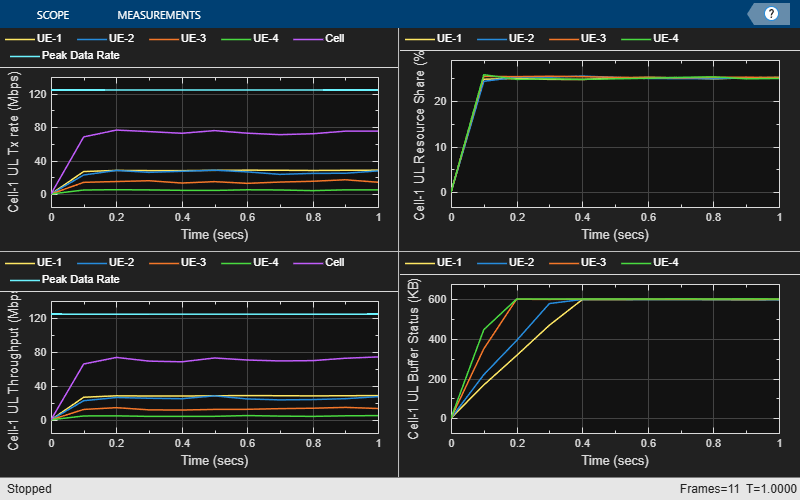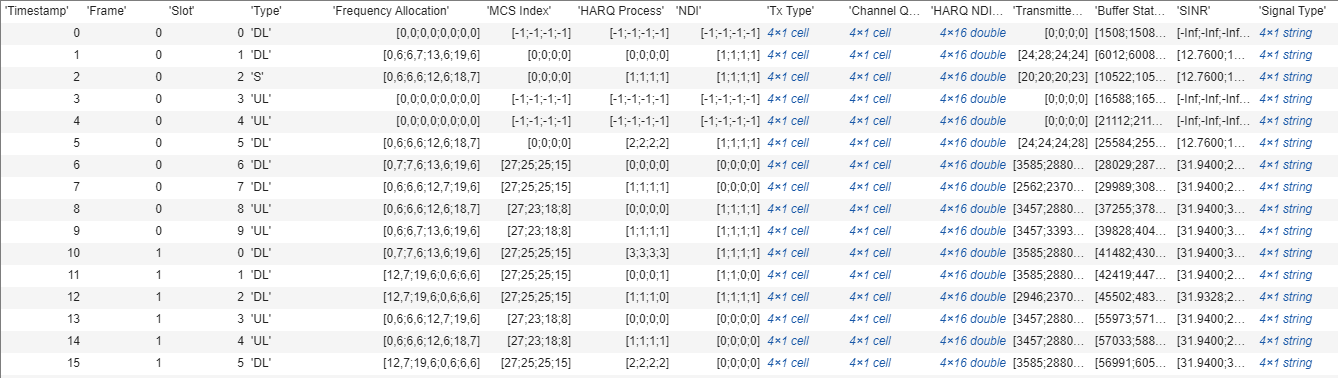### Simulation Visualization

The example shows four runtime visualization:

### Simulation Logs

The example saves the simulation parameters and logs as MAT-files for post-simulation analysis and visualization. The example saves the simulation parameters in a MAT-file with the file name as the value of configuration parameter, `simParameters.ParametersLogFile`. It also saves the per-time-step logs, scheduling assignment logs, and BLER logs in a MAT-file named as value of `simParameters.SimulationLogFile`. After the simulation, open the file to load `DLTimeStepLogs`, `SchedulingAssignmentLogs` in the workspace.

Time step logs: For details of log format, see the "Simulation Logs" section of the NR PUSCH FDD Scheduling example.

Scheduling assignment logs: Information about all the scheduling assignments. For details about the log format, see the "Simulation Logs" section of the NR FDD Scheduling Performance Evaluation example.

Block Error Rate logs: Block error information observed in the DL direction. For details of log format, see the 'Simulation Logs' section in the NR Cell Performance Evaluation with Physical Layer Integration example.

At the end of the simulation, the example compares the achieved value for system performance indicators against their theoretical peak values (considering zero overheads). Performance indicators displayed are: achieved data rate, achieved spectral efficiency and BLER observed for UEs. The peak value calculations are as per 3GPP TR 37.910.

```simulationLogs = cell(1,1); if(simParameters.DuplexMode == 0) % FDD logInfo = struct('DLTimeStepLogs',[], 'SchedulingAssignmentLogs',[],'BLERLogs',[]); else logInfo = struct('TimeStepLogs',[], 'SchedulingAssignmentLogs',[],'BLERLogs',[]); end dlStats = getPerformanceIndicators(simSchedulingLogger); [logInfo.BLERLogs, logInfo.AvgBLERLogs] = getBLERLogs(simPhyLogger); % Block Error rate logs fprintf('\nPeak DL throughput: %0.2f Mbps. Achieved average DL Throughput: %0.2f Mbps', dlStats(1, 1), dlStats(2, 1));```
```Peak DL throughput: 129.40 Mbps. Achieved average DL Throughput: 52.01 Mbps ```
`fprintf('\nAchieved average DL Goodput: %0.2f Mbps', dlStats(5, 1));`
```Achieved average DL Goodput: 51.95 Mbps ```
`fprintf('\nPeak DL spectral efficiency: %0.2f bits/s/Hz. Achieved average DL spectral efficiency: %0.2f bits/s/Hz', dlStats(3, 1), dlStats(4, 1));`
```Peak DL spectral efficiency: 12.94 bits/s/Hz. Achieved average DL spectral efficiency: 5.20 bits/s/Hz ```
`disp(['Block error rate for each UE in the downlink direction: [' num2str(round(logInfo.AvgBLERLogs(:, 1)', 2)) ']']);`
```Block error rate for each UE in the downlink direction: [0.01 0 0 0] ```

You can get a post-simulation visualization of logs by running the script NRPostSimVisualization. For more details about the options to run this script, see the NR FDD Scheduling Performance Evaluation example.

```% Read the logs and save them in MAT-files if(simParameters.DuplexMode == 0) % FDD logInfo.DLTimeStepLogs = getSchedulingLogs(simSchedulingLogger); else % TDD logInfo.TimeStepLogs = getSchedulingLogs(simSchedulingLogger); end logInfo.SchedulingAssignmentLogs = getGrantLogs(simSchedulingLogger); % Scheduling assignments log simulationLogs{1} = logInfo; save(simParameters.ParametersLogFile, 'simParameters'); % Save simulation parameters in a MAT-file save(simParameters.SimulationLogFile, 'simulationLogs'); % Save simulation logs in a MAT-file```

### Further Exploration

You can use this example to further explore custom scheduling.

#### Custom scheduling

You can modify the existing scheduling strategy to implement a custom strategy. Follow the steps in 'Further Exploration' section of the NR FDD Scheduling Performance Evaluation example, which explains the steps to write a custom scheduling strategy for single-input single-output (SISO) configuration. Additionally, MIMO configuration appends more fields to the scheduling assignment structure. Populate the fields of scheduling assignments with values for precoding matrix, number of layers as per your custom scheduling strategy. For more information about the information fields of a scheduling assignment, see the description of the `scheduleDLResourcesSlot` function in the hNRScheduler.m helper file.

The scheduler in the example selects the rank and precoding matrix which a UE reports in the CSI. You can also customize this behavior to select any rank and precoding matrix by overriding the function `selectRankAndPrecodingMatrix` in your custom scheduler. For more details, see the description of the `selectRankAndPrecodingMatrix` function in the hNRScheduler.m helper file

 3GPP TS 38.104. “NR; Base Station (BS) radio transmission and reception.” 3rd Generation Partnership Project; Technical Specification Group Radio Access Network.

 3GPP TS 38.214. “NR; Physical layer procedures for data.” 3rd Generation Partnership Project; Technical Specification Group Radio Access Network.

 3GPP TS 38.321. “NR; Medium Access Control (MAC) protocol specification.” 3rd Generation Partnership Project; Technical Specification Group Radio Access Network.

 3GPP TS 38.322. “NR; Radio Link Control (RLC) protocol specification.” 3rd Generation Partnership Project; Technical Specification Group Radio Access Network.

 3GPP TS 38.323. “NR; Packet Data Convergence Protocol (PDCP) specification.” 3rd Generation Partnership Project; Technical Specification Group Radio Access Network.

 3GPP TS 38.331. “NR; Radio Resource Control (RRC) protocol specification.” 3rd Generation Partnership Project; Technical Specification Group Radio Access Network.

 3GPP TR 37.910. “Study on self evaluation towards IMT-2020 submission.” 3rd Generation Partnership Project; Technical Specification Group Radio Access Network.